# College Physics : Spring Potential Energy and Other Potential Energy

## Example Questions

### Example Question #1 : Spring Potential Energy And Other Potential Energy

Suppose that an object of massis travelling towards a massless spring with a velocity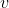. If the maximum displacement of the spring is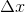, which of the following gives an expression for the spring constant,?

Note: Assume that there is no friction.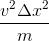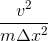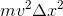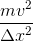Explanation:

For this question, we're told than an object is travelling with a certain velocity towards a spring. After colliding with the spring and causing it to undergo a maximum displacement, we're asked to provide an expression for the spring constant.

In order to answer this question, we'll need to consider the energy of the object and the spring during the process described. First, the object is moving with a certain velocity towards the spring. Thus, the object has kinetic energy. When the object collides with the spring and displaces the spring to its maximum displacement, there will be a brief instant where the object has completely stopped moving. At this point, all of the block's kinetic energy will have been transferred into the spring in the form of potential energy. With this information in mind, we can equate the two forms of energy.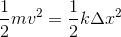Now, we can rearrange terms in order to isolate.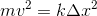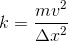### All College Physics Resources